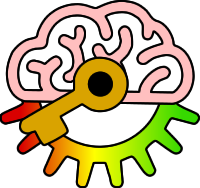# Y8

2j: Use logic gates to construct simple logic circuits.

Keywords:Test your self on these keywords and definitions using the games below or
KeywordDefinition
ANDLogic gate where the output is only On if both inputs are On
inputvalue that goes into a logic gate
Logic gateWay of combining On/Off values logically (AND, OR or NOT)
NOTLogic gate where the output is the opposite of the input
ORLogic gate where the output is On if either or both inputs are On
outputvalue that comes out as the result of a logic gate
Keyword games:
Resources: